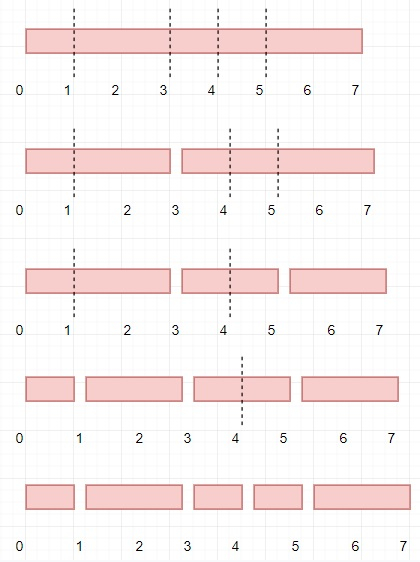# Program to find minimum cost to cut a stick in Python

Suppose we have a value n and an array called cuts. Consider there is a wooden stick of length n units. The stick is labelled from 0 to n. Here cuts[i] represents a position where we can cut. We should perform the cuts in order, but we can change the order of the cuts as we want. Here the cost of one cut is the size of the stick to be cut, and the total cost is the sum of costs of all cuts. We have to find the minimum total cost of the cuts.

So, if the input is like n = 7, cuts = [5,1,4,3], then the output will be 16 because if we order them like [3,5,1,4], then at first cut at 3 from length of 7, so cost is 7, then we have two parts of length 3 and 4, then cut at 5, so cost is 4, till now total is 7+4=11, then cut at 4 from stick size 2, so total cost 7+4+2 = 13, then finally cut at 3 so cost is 3, and final cost 7+4+2+3 = 16.To solve this, we will follow these steps −

• cuts := a list where elements in cuts are in sorted order, and insert 0 at beginning and n at end

• m := size of cuts

• cost := make a square matrix of size m x m and fill with 0

• for k in range 2 to m - 1, do

• for i in range 0 to m - 1, do

• j := i + k

• if j >= m, then

• go for next iteration

• cost[i, j] = (cuts[j] - cuts[i]) + minimum of (cost[i, s] + cost[s, j] for all s in range(i+1, j-1))

• return cost[0, m-1]

## Example

Let us see the following implementation to get better understanding

def solve(n, cuts):
cuts =  + sorted(cuts) + [n]
m = len(cuts)
cost = [*m for _ in range(m)]

for k in range(2, m):
for i in range(m):
j = i + k
if j >= m:
continue
cost[i][j] = (cuts[j]-cuts[i]) + min(cost[i][s] + cost[s][j] for s in range(i+1, j))

return cost[m-1]

n = 7
cuts = [5,1,4,3]
print(solve(n, cuts))

## Input

7, [5,1,4,3]


## Output

16